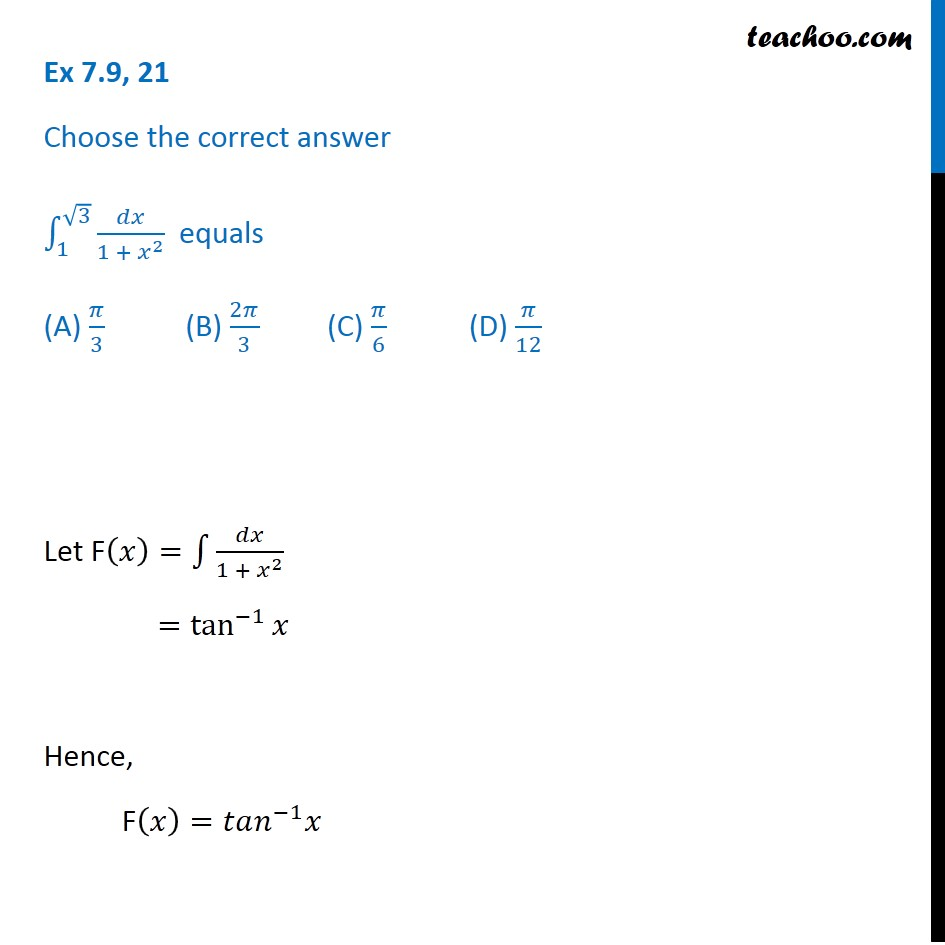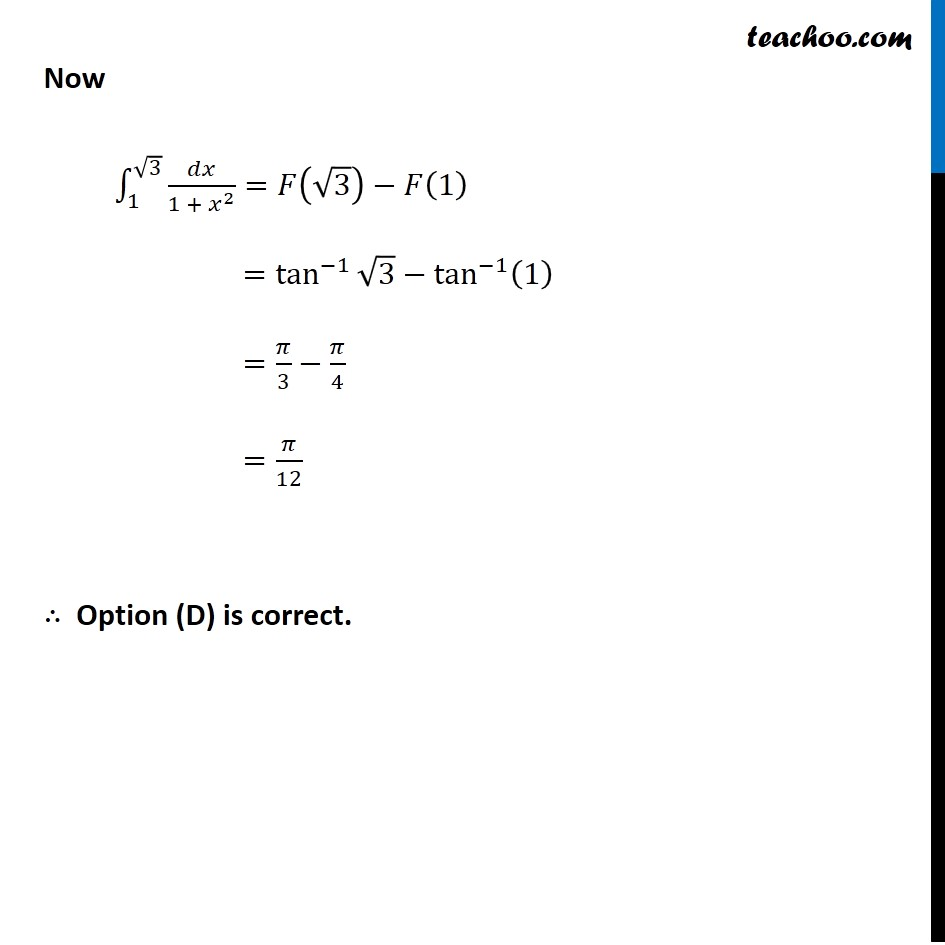Integration Full Chapter Explained - Integration Class 12 - Everything you need1. Chapter 7 Class 12 Integrals
2. Concept wise
3. Definite Integration - By Formulae

Transcript

Ex 7.9, 21 Choose the correct answer ∫_1^(√3)▒𝑑𝑥/(1 + 𝑥^2 ) equals (A) 𝜋/3 (B) 2𝜋/3 (C) 𝜋/6 (D) 𝜋/12 Let F(𝑥)=∫1▒𝑑𝑥/(1 + 𝑥^2 ) =tan^(−1)⁡𝑥 Hence, F(𝑥)=𝑡𝑎𝑛^(−1) 𝑥 Now ∫_1^(√3)▒〖𝑑𝑥/(1 + 𝑥^2 )=𝐹(√3)−𝐹(1) 〗 =tan^(−1)⁡〖√3−tan^(−1)⁡(1) 〗 =𝜋/3−𝜋/4 =𝜋/12 ∴ Option (D) is correct.

Definite Integration - By Formulae

About the AuthorDavneet Singh
Davneet Singh is a graduate from Indian Institute of Technology, Kanpur. He has been teaching from the past 9 years. He provides courses for Maths and Science at Teachoo.